Test: Circuit Theorems - 1

# Test: Circuit Theorems - 1 - Electrical Engineering (EE)

Test Description

## 10 Questions MCQ Test Topicwise Question Bank for Electrical Engineering - Test: Circuit Theorems - 1

Test: Circuit Theorems - 1 for Electrical Engineering (EE) 2023 is part of Topicwise Question Bank for Electrical Engineering preparation. The Test: Circuit Theorems - 1 questions and answers have been prepared according to the Electrical Engineering (EE) exam syllabus.The Test: Circuit Theorems - 1 MCQs are made for Electrical Engineering (EE) 2023 Exam. Find important definitions, questions, notes, meanings, examples, exercises, MCQs and online tests for Test: Circuit Theorems - 1 below.
Solutions of Test: Circuit Theorems - 1 questions in English are available as part of our Topicwise Question Bank for Electrical Engineering for Electrical Engineering (EE) & Test: Circuit Theorems - 1 solutions in Hindi for Topicwise Question Bank for Electrical Engineering course. Download more important topics, notes, lectures and mock test series for Electrical Engineering (EE) Exam by signing up for free. Attempt Test: Circuit Theorems - 1 | 10 questions in 30 minutes | Mock test for Electrical Engineering (EE) preparation | Free important questions MCQ to study Topicwise Question Bank for Electrical Engineering for Electrical Engineering (EE) Exam | Download free PDF with solutions
 1 Crore+ students have signed up on EduRev. Have you?
Test: Circuit Theorems - 1 - Question 1

### Superposition theorem is applicable only to network that are

Test: Circuit Theorems - 1 - Question 2

### In any lumped network with elements in b  branches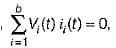for all holds good  according to

Detailed Solution for Test: Circuit Theorems - 1 - Question 2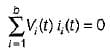means the total sum of instantenous power consumed in various elements in the branches of a network is zero, which is the statement of Tellegen’s theorem.

Test: Circuit Theorems - 1 - Question 3

### The Theven's equivalent resistance to the left of AB in figure given below is given by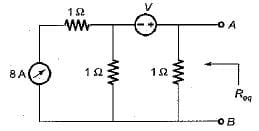Detailed Solution for Test: Circuit Theorems - 1 - Question 3

For finding the Thevenin’s equivalent resistance, 8 A current source will be open circuited while v volt voltage source will be short circuited.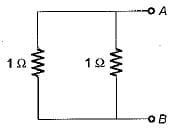Hence,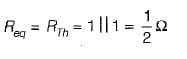Test: Circuit Theorems - 1 - Question 4

For which of the following, Thevenin’s and Norton’s equivalents can’t be developed?

Test: Circuit Theorems - 1 - Question 5

The value of Z22 (Ω) for the circuit shown below is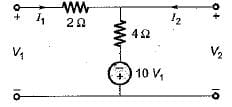Detailed Solution for Test: Circuit Theorems - 1 - Question 5

Applying KVL, we get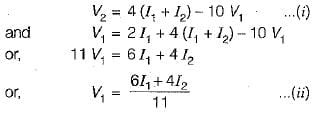Putting the value of V1 from equation (ii) into equation (i), we get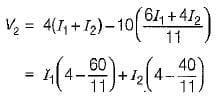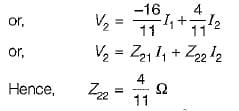Test: Circuit Theorems - 1 - Question 6

In which of the following it is not desired to attain the condition of maximum power transfer?

Detailed Solution for Test: Circuit Theorems - 1 - Question 6

Computer circuits

Explanation:
The Maximum Power Transfer Theorem states that the maximum amount of power will be transferred from the source to the load when the load resistance is equal to the source resistance. This is important in electronic and electric circuits, as well as communicational circuits, where the primary goal is to transfer power efficiently.

However, in computer circuits, the primary goal is not to transfer power but to process information, perform calculations, and support various functionalities. In these circuits, the primary focus is on the efficient processing of information and minimizing power consumption. Maximum power transfer is not a key objective in computer circuits, as it can lead to increased power consumption and increased heat generation, which is undesirable for computer systems.

Test: Circuit Theorems - 1 - Question 7

A simple equivalent circuit of the two terminal network shown in figure below is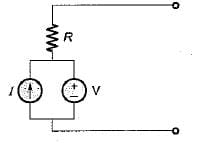Detailed Solution for Test: Circuit Theorems - 1 - Question 7

For the given circuit, Req across the given terminals = R.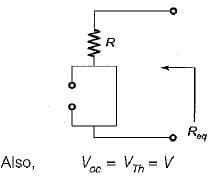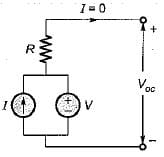Test: Circuit Theorems - 1 - Question 8

For the circuit shown below, a resistance R is to be connected across an active network, it is given that when

(i) R =∞, V = 5 volts

(ii) R = 0, current output of the active source is 2.5 A.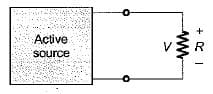What would be the current through tfwhen the value of R is 5 Ω?

Detailed Solution for Test: Circuit Theorems - 1 - Question 8

When R = ∞, Voc= V = 5 volts
When R = 0 , ISC = 2 .5 A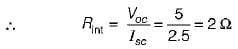Hence, the Thevenin's equivalent circuit will be drawn as shown below.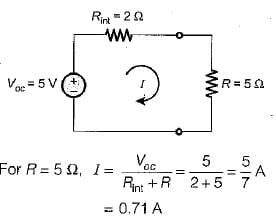Test: Circuit Theorems - 1 - Question 9

A generator of internal impedance I ZgI delivers maximum power to a load impedance Zp , only if

Test: Circuit Theorems - 1 - Question 10

In the circuit shown below, the current through the 5Ω resistor is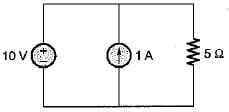Detailed Solution for Test: Circuit Theorems - 1 - Question 10

An ideal voltage source is never short circuited. Hence, we should not use superposition theorem. The voltage across the 5Ω resistor will be 10 volts. Hence, current through the 5 Ω resistor

=10/5 = 2 A

## Topicwise Question Bank for Electrical Engineering

211 tests
Information about Test: Circuit Theorems - 1 Page
In this test you can find the Exam questions for Test: Circuit Theorems - 1 solved & explained in the simplest way possible. Besides giving Questions and answers for Test: Circuit Theorems - 1, EduRev gives you an ample number of Online tests for practice

## Topicwise Question Bank for Electrical Engineering

211 tests(Scan QR code)